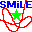SMILE  v2.5 Schwarzschild Modelling Interactive expLoratory Environment
measureshape.cpp File Reference

Tool: Measure the shape (axis ratio) of an N-body snapshot as a function of radius. More...

#include <math.h>
#include <stdlib.h>
#include <stdinc.h>
#include <getparam.h>
#include <io_nemo.h>
#include <snapshot/snapshot.h>
#include <vector>
#include <algorithm>
#include <iostream>
#include <gsl/gsl_math.h>
#include <gsl/gsl_eigen.h>
#include <gsl/gsl_roots.h>
#include <gsl/gsl_errno.h>
#include "utils.h"
Include dependency graph for measureshape.cpp:Functions

int main (int argc, char *argv[])

Variables

string defv []

string usage =const_cast<char*>("Measure axis ratios as a function of radius. Two methods: equidensity ellipsoids axis ratio (if density is decreasing with radius) or inertia tensor. Snapshot must be centered on the density maximum or results will be rubbish.")

Detailed Description

Tool: Measure the shape (axis ratio) of an N-body snapshot as a function of radius.

Date
2008-2015

Two methods for shape determination are available:

• Equidensity ellipsoid (provided that density is a decreasing function of radius); this method is used in Athanassoula & Misiriotis(2002) and requires that density information be present in the snapshot.
• Inertia tensor of particles with iteratively determined axes (see Zemp et al.(2011) for an extended discussion on variations of this method). The axes are determined from the inertia tensor of particles within ellipsoidal volume, with the ellipsoid itself using the axes determined on the previous iteration, until it converges.

The program processes input snapshots in NEMO format (a file may contain snapshots at multiple times), which should be centered but not necessarily rotated to match principal planes.

The axis ratios are computed for several bins in radius, either using all particles inside the radius of this bin (i.e. including those in all previous bins), or in the current bin only. Output may be presented either in the short or the long forms:

• for the short format, each line contains the time and 2*nbins values for b/a and c/a axes, one line per snapshot at a given time;
• for the long one, each snapshot is displayed in nbins lines, with the radius, mass(r), b/a and c/a, and optionally orientations of the axes of the inertia tensor.

Variable Documentation

 string defv[]
Initial value:
=
{
const_cast<char*>("in=???\n input snapshot"),
const_cast<char*>("out=\n optional output file for rectified snapshot"),
const_cast<char*>("nbins=20\n number of bins in which to calculate the axis ratios"),
const_cast<char*>("dens=f\n choice of method: equidensity ellipsoid (t) or inertia tensor (f)"),
const_cast<char*>("cutoff=0.999\n last bin encompasses this fraction of total mass"),
const_cast<char*>("center=t\n shift snapshot to the center (defined as median of the particle coordinates)"),
const_cast<char*>("minbin=0\n fraction of mass in the first bin (relative to Mtot*cutoff; by default = 1/nbins, may be smaller in which case bins will be spaced quasi-exponentially in enclosed mass)"),
const_cast<char*>("cumul=t\n each bin contains all stars within radius (t) or only those not in previous bins (f); the latter option should be used with caution since it may not always converge"),
const_cast<char*>("compact=f\n print all data at given time in one line (t) or in multiple (one per radius)"),
const_cast<char*>("angles=f\n also print the orientations of the major and minor axes (angles between xy-component of major axis and X axis, and angle between minor axis and Z axis)"),
const_cast<char*>("VERSION=2.5\n Jun 2008 Rubens, 2010-2015 EV"),
NULL
}